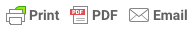### 3 Essential Trading Indicators Which Are Rocking In 2020

Posted July 7, 2020 by rafatsunny

There are many different types of indicators, and they are widely used by day traders, swing traders, and sometimes even longer-term investors.

1. Relative Strength Index (RSI)

The RSI is a momentum indicator that shows whether an asset is overbought or oversold. It does this by measuring the magnitude of recent price changes (the standard-setting is the previous 14 periods — so 14 days, 14 hours, etc.).

The data is then displayed as an oscillator that can have a value between 0 and 100.
Since the RSI is a momentum indicator, it shows the rate (momentum) at which the price is changing.
This means that if momentum is increasing while the price is rising, the uptrend is strong, and more and more buyers are stepping in.

In contrast, if momentum is decreasing while the price is rising, it may show that sellers soon might take control over the market.

A traditional interpretation of the RSI is that when it’s over 70, the asset is overbought, and when it’s under 30, it is oversold.

As such, extreme values may indicate an impending trend reversal or pullback.

Even so, it might be best not to think about these values as direct buy or sell signals. As with much other technical analysis (TA) techniques, the RSI may provide false or misleading signals, so it’s always useful to consider other factors before entering a trade.

2. Moving Average (MA)

A moving average smooths out price action by filtering out market noise and highlighting the direction of the trend. As it’s based on past price data, it’s a lagging indicator.

The two most commonly used moving averages are the simple moving average (SMA or MA), and the exponential moving average (EMA).

The SMA is plotted by taking price data from the defined period and producing an average.

For example, the 10-day SMA is plotted by calculating the average price over the last 10 days. The EMA, on the other hand, is calculated in a way that gives more weight to recent price data. This makes it more reactive to recent price action.

As mentioned, the moving average is a lagging indicator. The longer the period, the greater the lag. As such, the 200-day SMA will react slower to recent price action than the 50-day SMA.

Traders often use the relationship of the price to specific moving averages to gauge the current market trend.
For example, if the price stays above the 200-day SMA for a prolonged period, the asset may be considered to be in a bull market by many traders.

Traders may also use moving average crossovers as buy or sell signals. For example, if the 100-day SMA crosses below the 200-day SMA, it may be considered a sell signal. But what exactly does this cross mean?

It indicates that the average price over the last 100 days is now below that of the last 200 days. The idea behind selling here is that short-term price movements are no longer following the uptrend, so the trend may be reversing.

3. Moving Average Convergence Divergence (MACD)

The MACD is used to determine the momentum of an asset by showing the relationship between two moving averages. It’s made up of two lines — the MACD line and the signal line.

The MACD line is calculated by subtracting the 26 EMA from the 12 EMA. This is then plotted over the MACD line’s 9 EMA — the signal line.

Many charting tools also often incorporate a histogram, which shows the distance between the MACD line and the signal line.

By looking for divergences between the MACD and the price action, traders might gain insight into the strength of the current trend.

For example, if the price is making a higher high, while the MACD is making a lower high, the market may be reversing soon.

What is the MACD telling us in this case? That price is increasing while momentum is decreasing, so there is a higher probability of a pullback or reversal occurring.

Traders may also use this indicator to look for crossovers between the MACD line and its signal line. For example, if the MACD line crosses above the signal line, that may suggest a buy signal.

Conversely, if the MACD line crosses below the signal line, that may indicate a sell signal.
The MACD is often used in combination with the RSI, as they both measure momentum, but by different factors. The assumption is that together they may give a more complete technical outlook on the market.DisclaimerReport Abuse